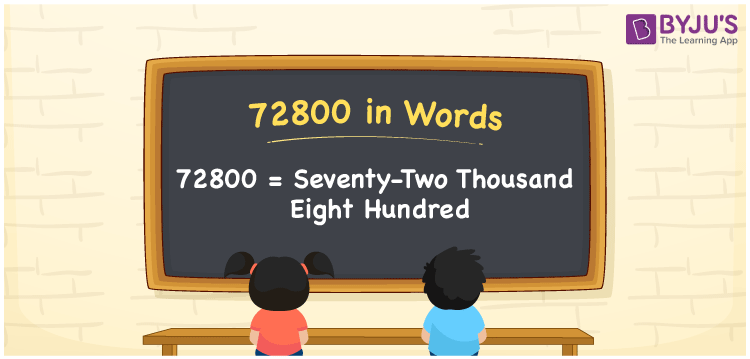We can write 72800 in words as Seventy-two thousand eight hundred. If you spent Rs. 72800 to assemble a partition in a house, you can say, “I spent Seventy-two thousand eight hundred rupees on assembling a partition in the house”. Also, we know that 72800 is a cardinal number since it denotes a specific quantity.

 72800 in words Seventy-two thousand eight hundred Seventy-two thousand eight hundred in Numbers 72800

## 72800 in English words

We generally express numbers in words using the English alphabet. Thus, we spell 72800 in English as “Seventy-two thousand eight hundred”.## How to Write 72800 in Words?

The number 72800 contains five digits so let’s make a place value chart with five columns to convert it into words, as shown below.

 Ten thousands Thousands Hundreds Tens Ones 7 2 8 0 0

Here, ones = 0, tens = 0, hundreds = 8, thousands = 2, ten thousands = 7.

By expanding these digits, we get;

7 × Ten thousand + 2 × Thousand + 8 × Hundred + 0 × Ten + 0 × One

= 7 × 10000 + 2 × 1000 + 8 × 100 + 0 × 10 + 0 × 1

= 70000 + 2000 + 800

= Seventy thousand + Two thousand + Eight hundred

= Seventy-two thousand eight hundred

Therefore, 72800 in words = Seventy-two thousand eight hundred.

72800 is a natural number that is the successor of 72799 and the predecessor of 72801.

72800 in words – Seventy-two thousand eight hundred

Is 72800 an odd number? – No

Is 72800 an even number? – Yes

Is 72800 a perfect square number? – No

Is 72800 a perfect cube number? – No

Is 72800 a prime number? – No

Is 72800 a composite number? – Yes

## Frequently Asked Questions on 72800 in Words

Q1

### Write the number 72800 in words.

We can write the number 72800 in words as Seventy-two thousand eight hundred.
Q2

### How to write Rs. 72800 in words on a cheque?

On a cheque, we generally write Rs. 72800 in words as Seventy-two thousand eight hundred rupees only.
Q3

### How to convert 72800 into words?

We can convert the number 72800 into words as follows: 72800 = 70000 + 2000 + 800 = Seventy thousand + two thousand + eight hundred = Seventy-two thousand eight hundred